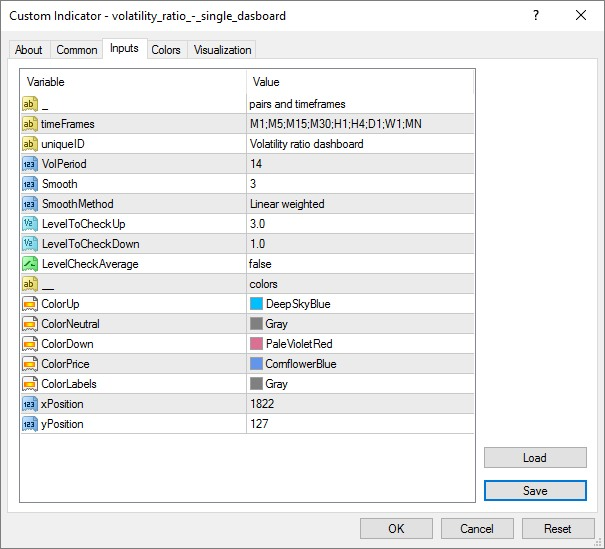Elite indicators :) - page 1346151210

Dimi.A:

Guys does any one know how to use the VOLATILITY RATIO DASHBOARD?

What does it do? And what do the pink colours mean?

Thanks guys.

Calculates the volatility as a ratio of true range to average true range. If that value is above the required level, then it uses the color for up. If that value is bellow the required level, then it uses the color for down. Otherwise it is using the color for neutral. And it does that for all the required time frames

PS: true range is a special case of average true range - it is an average true range with period 1. And since it calculates the true range only for the current bar, then the ratio of true range to average true range can vary wildly (having large values) in the times of elevated volatility168
Dimi.A

Calculates the volatility as a ratio of true range to average true range. If that value is above the required level, then it uses the color for up. If that value is bellow the required level, then it uses the color for down. Otherwise it is using the color for neutral. And it does that for all the required time frames

PS: true range is a special case of average true range - it is an average true range with period 1. And since it calculates the true range only for the current bar, then the ratio of true range to average true range can vary wildly (having large values) in the times of elevated volatility

Thanks Mladen. So if the colour for down is lit up on all pairs, does that mean the volatility is "low" at this stage?151210

Dimi.A:
Thanks Mladen. So if the colour for down is lit up on all pairs, does that mean the volatility is "low" at this stage?
Yes.168
Dimi.A
Yes.

Cheers. What would be the ideal settings for it to be more "sensitive"? As in, if there is a movement in the 1M chart of more than 5 pips, is there anything I can change in the settings to show high volatility for this? Set the Volume Period to 1 perhaps?151210

Dimi.A:

Cheers. What would be the ideal settings for it to be more "sensitive"? As in, if there is a movement in the 1M chart of more than 5 pips, is there anything I can change in the settings to show high volatility for this? Set the Volume Period to 1 perhaps?

Dimitri

Try setting the level up to smaller value and VolPeriod to greater value (if you set the VolPeriod to 1, then it will always be equal to true range - it will never change the color)168
Dimi.A

Dimitri

Try setting the level up to smaller value and VolPeriod to greater value (if you set the VolPeriod to 1, then it will always be equal to true range - it will never change the color)

Will do. Thank you bro.94

Would you please add some type of filter to this indicator ?

Files:151210

bayuveneo:
Would you please add some type of filter to this indicator ?

Here is this one first (usual set of 3 filter types)

Files:168
Dimi.A
Yes.

Mladen is there any way we can put this indicator "Vertex Alerts NMC" on the main chart window (like volume is displayed) please?

Files:151210

Dimi.A:

Mladen is there any way we can put this indicator "Vertex Alerts NMC" on the main chart window (like volume is displayed) please?

Dimi

We can not, sorry. That way of drawing indicators is not available in metatrader